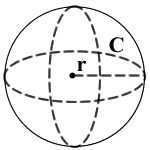## Sphere Volume Surface Area calculator

 r = Units: A = 0 V = 0 C = 0

r = radius, V = volume, A = surface area, C = circumference

» Show Work

A sphere is a solid geometrical object, such as basketball PingPong is the shape. For more information to find the various properties of a sphere, you must know the some values of the sphere like radius volume and so on.

The calculator to find the volume, surface area, radius, and circumference of a sphere using the formula below.### SphereFormulas

Sphere Volume = (4/3) π r3

Sphere Surface Area = 4 π r2

The Area and Volume of a Sphere can be calculated in different units by multiplying respective value to the resulting value. These are all the below values are used to convert the units into different units

For example, r = 2,

1. To calculate A, use the following formula: 4πr2. When you substitute r for its value, you get 4π(22), which equals 50.26548 m2.
2. To calculate V, use the following formula: (4/3)πr3. When you substitute r for its value, you get (4/3)π(23), which equals 33.51032 m3.
3. To calculate C, use the following formula: 2πr. When you substitute r for its value, you get 2π(2), which equals 12.56637 m.

Thinkcalculator.com provides you helpful and handy calculator resources.